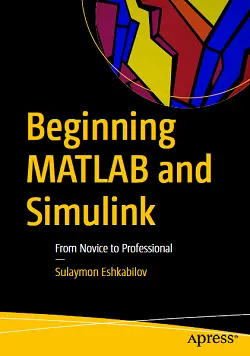# Beginning MATLAB and Simulink### eBook Details:

• Paperback: 524 pages
• Publisher: WOW! eBook; 1st edition (November 29, 2019)
• Language: English
• ISBN-10: 1484250605
• ISBN-13: 978-1484250600

### eBook Description:

Beginning MATLAB and Simulink: From Novice to Professional

Employ essential and hands-on tools and functions of the MATLAB and Simulink packages, which are explained and demonstrated via interactive examples and case studies. This book contains dozens of simulation models and solved problems via m-files/scripts and Simulink models which help you to learn programming and modeling essentials. You’ll become efficient with many of the built-in tools and functions of MATLAB/Simulink while solving engineering and scientific computing problems.

What You Will Learn

• Get started using MATLAB and Simulink
• Carry out data visualization with MATLAB
• Gain the programming and modeling essentials of MATLAB
• Build a GUI with MATLAB
• Work with integration and numerical root finding methods
• Apply MATLAB to differential equations-based models and simulations
• Use MATLAB for data science projects

Beginning MATLAB and Simulink explains various practical issues of programming and modelling in parallel by comparing MATLAB and Simulink. After reading and using this book, you’ll be proficient at using MATLAB and applying the source code from the book’s examples as templates for your own projects in data science or engineering.

### 1 Response

1. May 2, 2021

[…] MATLAB Recipes: A Problem-Solution Approach, 2nd Edition: The first developer reference that covers up to MATLAB 2020b […]